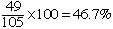Here is the question; If sales for jan 2001 are 154 vs 105 for 2000 - the increase total presented to my office is +46% - how does that compute. Please give me the equation. I am a middle aged business man. Thanks Hi Bob, Since Jan 2000 the sales have increased 154 - 105 = 49 units. Percentage increase is the increase, as a percentage of the sales there were at the beginning of the period. At the beginning of the period, Jan 2000 the sales were 105. Hence the percentage increase isCheers, Penny Go to Math Central